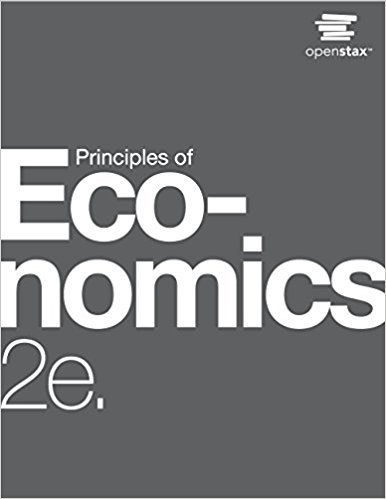×
Get Full Access to Principles Of Economics - 2 Edition - Chapter 6 - Problem 2
Get Full Access to Principles Of Economics - 2 Edition - Chapter 6 - Problem 2

×

# Solved: Take Jeremys total utility information in Exercise 6.1, and use the marginalISBN: 9781947172364 471

## Solution for problem 2 Chapter 6

Principles of Economics | 2nd Edition

• Textbook Solutions
• 2901 Step-by-step solutions solved by professors and subject experts
• Get 24/7 help from StudySoup virtual teaching assistantsPrinciples of Economics | 2nd Edition

4 5 1 340 Reviews
23
3
Problem 2

Take Jeremys total utility information in Exercise 6.1, and use the marginal utility approach to confirm the choice of phone minutes and round trips that maximize Jeremys utility.

Step-by-Step Solution:
Step 1 of 3

Practice Questions for Stocks and Bonds and Explanation ( Part 2 ) Explaining Important Most important 11) The yield to maturity on a discount bond is : a. equal to the coupon rate. b. greater than the coupon rate. c. less than the coupon rate Answer B : discount bond means they need to pay you more, when we have the yield to maturity > Coupon rate => price bond < par value 12) The dividend growth model can be used to value the stock of firms which pay which type ofdividends I. constant annual dividend II. annual dividend with a constant increasing rate of growth III. zero dividend A. I only B. II only C. I and II only D. I, II, and III only Answer C : Based on the basic formula : Po = Do ( 1 + g) / (R-g) = D1 / (R-g) 13) Which one of the foll

Step 2 of 3

Step 3 of 3

##### ISBN: 9781947172364

Since the solution to 2 from 6 chapter was answered, more than 222 students have viewed the full step-by-step answer. Principles of Economics was written by and is associated to the ISBN: 9781947172364. This textbook survival guide was created for the textbook: Principles of Economics, edition: 2. The answer to “Take Jeremys total utility information in Exercise 6.1, and use the marginal utility approach to confirm the choice of phone minutes and round trips that maximize Jeremys utility.” is broken down into a number of easy to follow steps, and 28 words. This full solution covers the following key subjects: . This expansive textbook survival guide covers 37 chapters, and 1291 solutions. The full step-by-step solution to problem: 2 from chapter: 6 was answered by , our top Business solution expert on 03/16/18, 04:24PM.

Unlock Textbook Solution